POINT CHARGE MODEL (PCM )
The point charge model is a simplified model of a crystal lattice in which ligands are presented as charged spheres with a uniform surface charge distribution that excludes the screening effects of the outer shell. This allows us to determine the Amn coefficients, which is equivalent to specifying the potential at a given point (i.e. in our case, the potential energy acting on the central ion). The  coefficients are introduced due to the fact that the: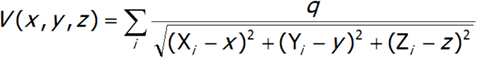where: Xi, Yi, Zi – ligand coordinates, X,y,z – position of the central ion electron. By expanding it into a Maclaurin series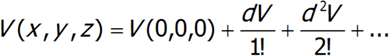we will  have reached an expansion whose coefficients are in line with our  Amn parameters. The potentials for the three basic symmetries will have the following form:

• The potential of charges in the corners of the square away from the plane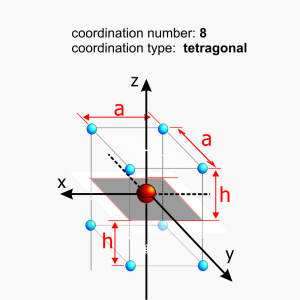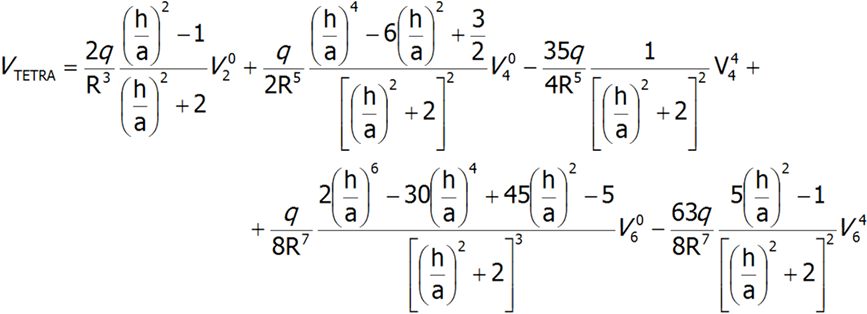Upon rotating the coordinate system by 45°, the signs of sections V44  and  V46  are altered

• The potential of charges located in the corners of a remote equilateral triangle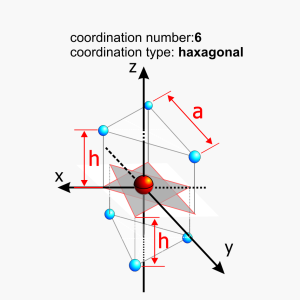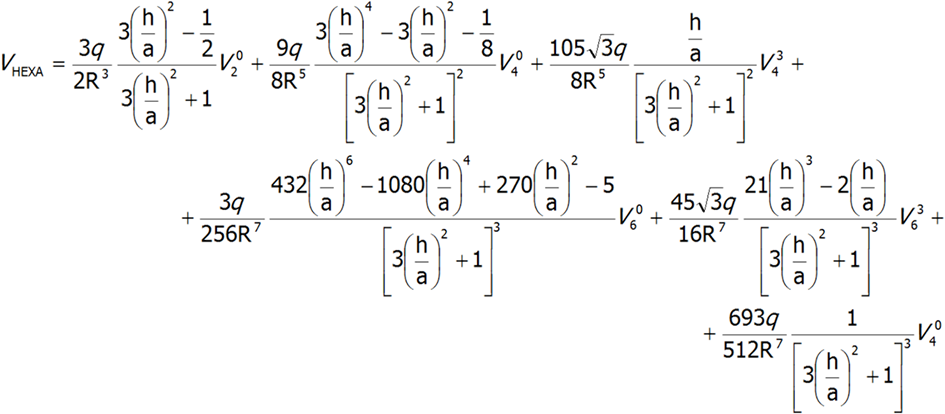Upon reflecting in the horizontal plane, the signs of sections V34  and  V36   are altered

• The axial potential of a point charge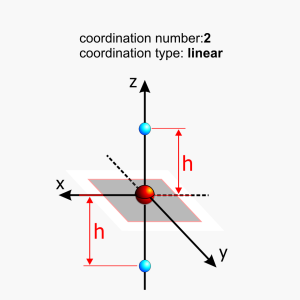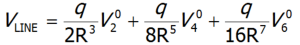By rotation and  superposition of these three components, we can compose the symmetry of the most highly symmetrical potentials carried by ligands in crystals.